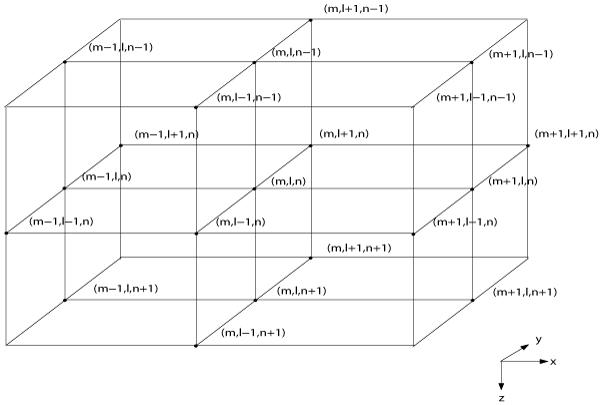A 19-Point Average-derivative Optimal Scheme

Our Group organises 3000+ Global Conferenceseries Events every year across USA, Europe & Asia with support from 1000 more scientific societies and Publishes 700+ Open Access Journals which contains over 50000 eminent personalities, reputed scientists as editorial board members.

###### A 19-Point Average-derivative Optimal Scheme

A 27-point optimal scheme for 3D frequency-domain scalar wave equation was recently developed. This scheme includes 9 optimization coefficients, and is relatively complicated. To simplify this scheme, a 19-point average-derivative optimal scheme for 3D frequency-domain scalar wave equation is constructed in this paper. This new 19-point scheme includes only 5 optimization coefficients, but maintains similar advantages of the 27-point optimal scheme. Compared to the classical 7-point scheme, the number of grid points per shortest wavelength is reduced from approximately 13 to approximately 4 by this 19-point optimal scheme for equal directional sampling intervals and unequal directional sampling intervals as well. Two numerical examples are presented to demonstrate the theoretical analysis.